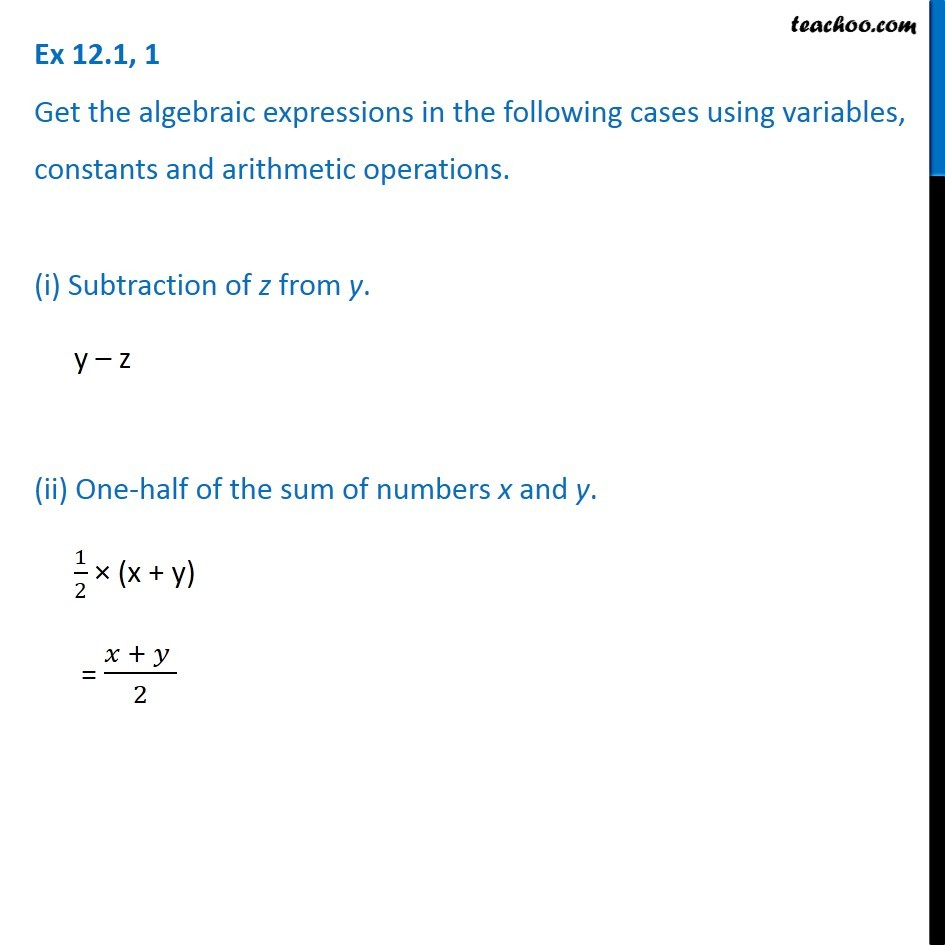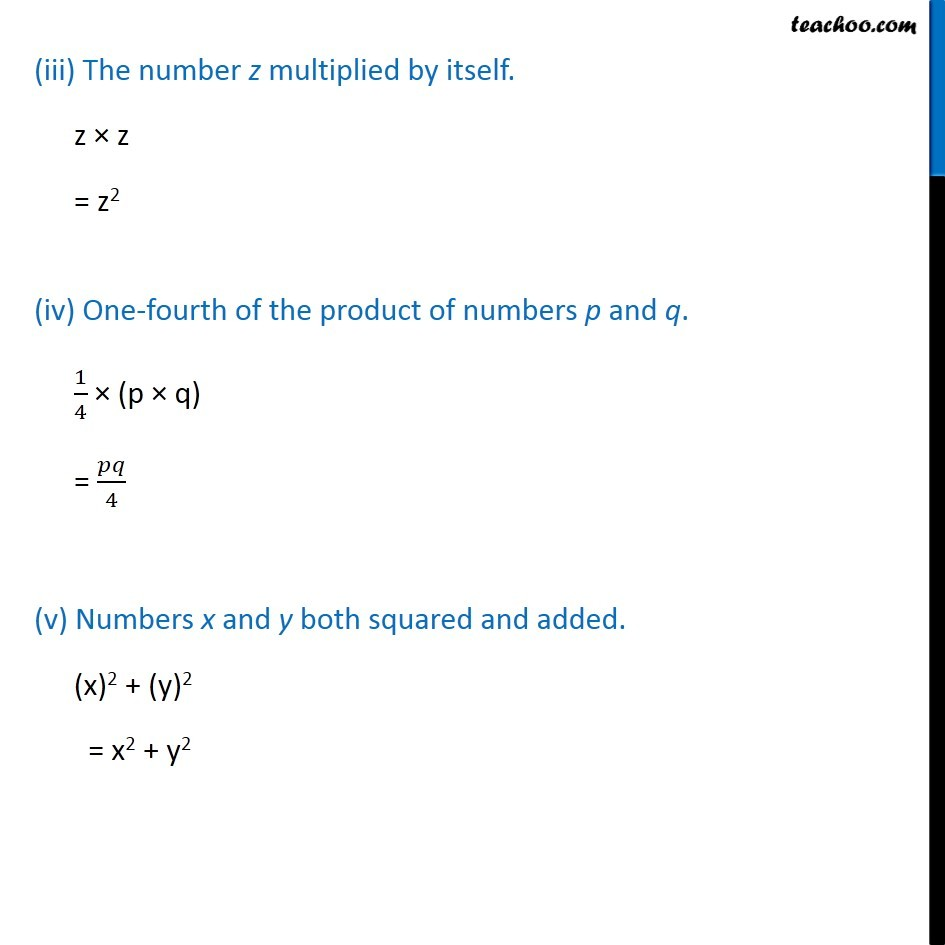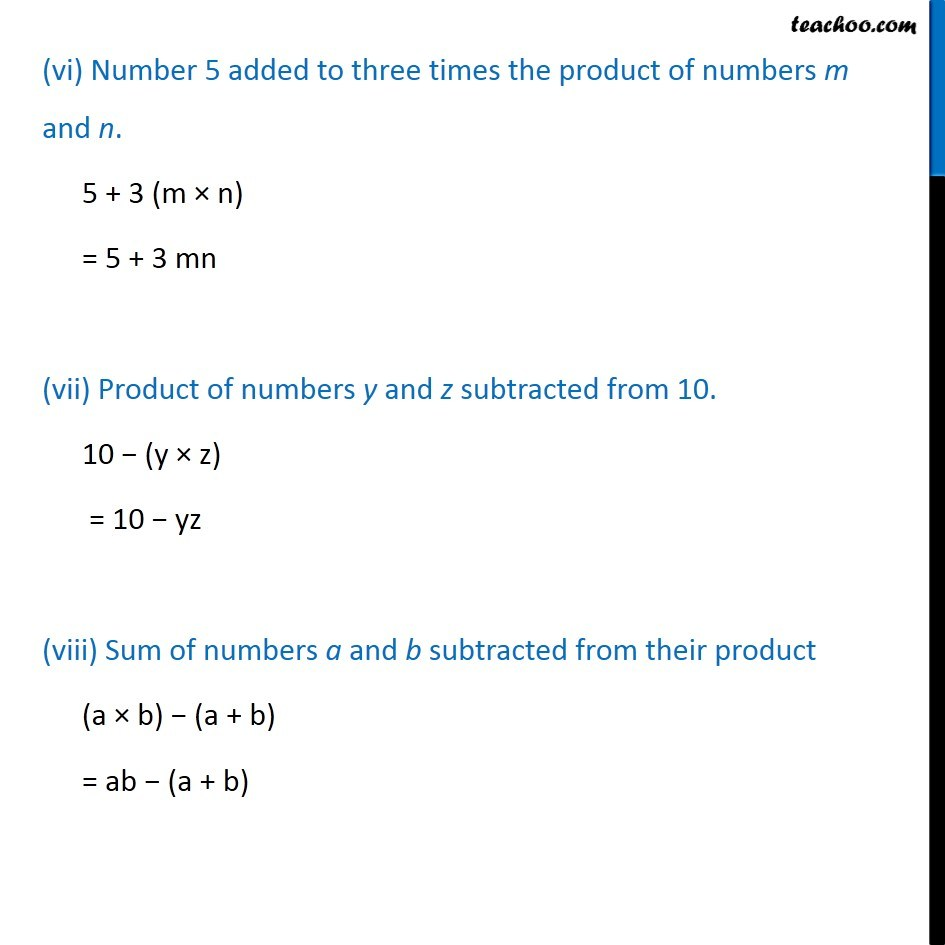Subscribe to our Youtube Channel - https://you.tube/teachoo

1. Chapter 12 Class 7 Algebraic Expressions
2. Concept wise
3. Definitions

Transcript

Ex 12.1, 1 Get the algebraic expressions in the following cases using variables, constants and arithmetic operations. (i) Subtraction of z from y. y – z (ii) One-half of the sum of numbers x and y. 1/2 × (x + y) = (𝑥 + 𝑦 )/2 (iii) The number z multiplied by itself. z × z = z2 (iv) One-fourth of the product of numbers p and q. 1/4 × (p × q) = 𝑝𝑞/4 (v) Numbers x and y both squared and added. (x)2 + (y)2 = x2 + y2 (vi) Number 5 added to three times the product of numbers m and n. 5 + 3 (m × n) = 5 + 3 mn (vii) Product of numbers y and z subtracted from 10. 10 − (y × z) = 10 − yz (viii) Sum of numbers a and b subtracted from their product (a × b) − (a + b) = ab − (a + b)

Definitions